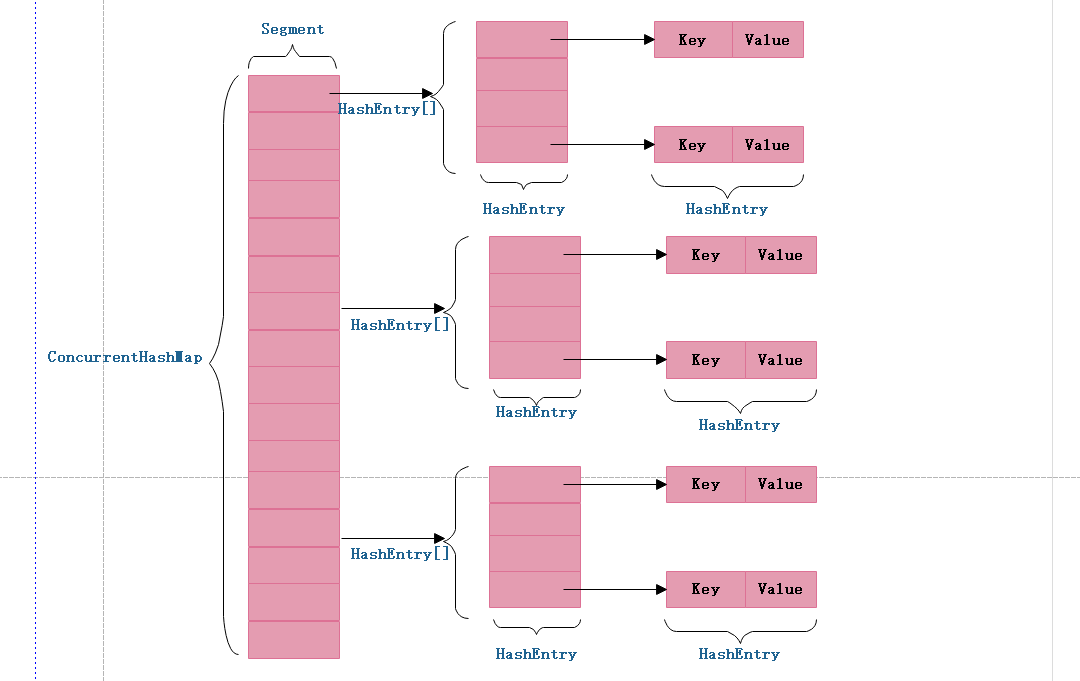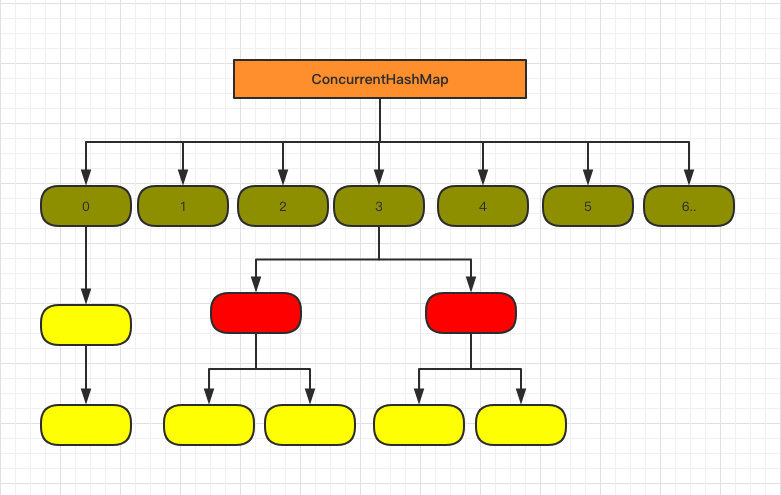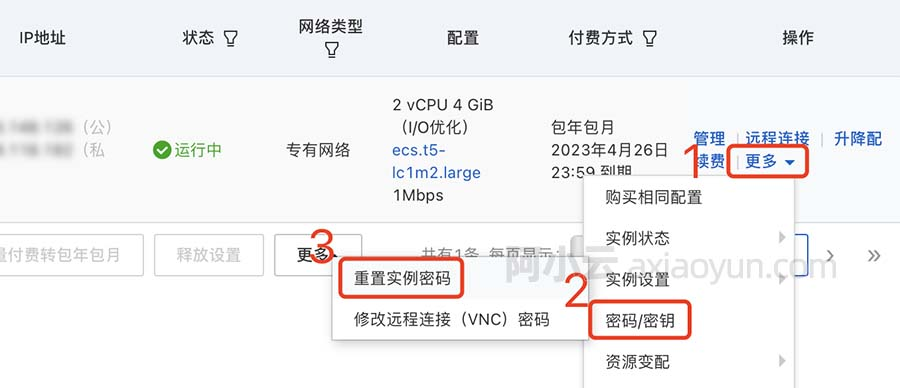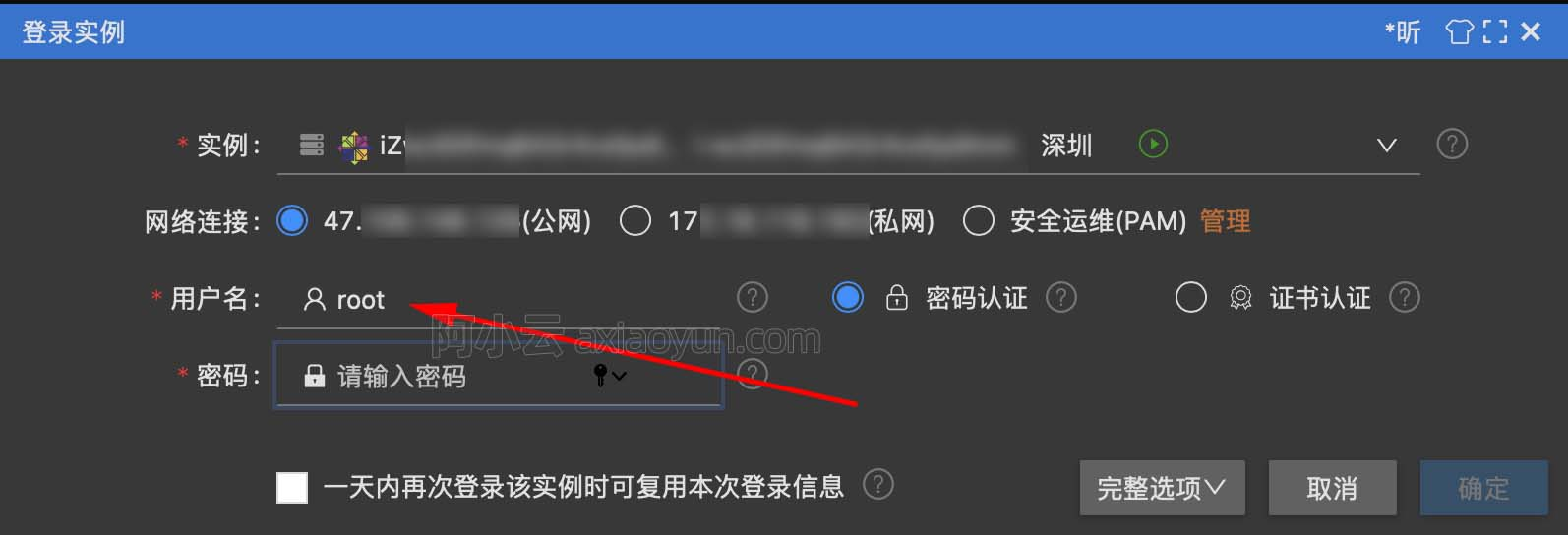Java并发编程笔记之ConcurrentHashMap原理探究

+关注继续查看

HashTable是一个线程安全的类，它使用synchronized来锁住整张Hash表来实现线程安全，即每次锁住整张表让线程独占，相当于所有线程进行读写时都去竞争一把锁，导致效率非常低下。ConcurrentHashMap可以做到读取数据不加锁，并且其内部的结构可以让其在进行写操作的时候能够将锁的粒度保持地尽量地小，允许多个修改操作并发进行，其关键在于使用了锁分离技术。它使用了多个锁来控制对hash表的不同部分进行的修改。ConcurrentHashMap内部使用段(Segment)来表示这些不同的部分，每个段其实就是一个小的Hashtable，它们有自己的锁。只要多个修改操作发生在不同的段上，它们就可以并发进行。

JDK1.7中的CouncurrentHashMap实现原理

ConcurrentHashMap 为了提高本身的并发能力，在内部采用了一个叫做 Segment 的结构，一个 Segment 其实就是一个类 Hash Table 的结构，Segment 内部维护了一个链表数组，我们用下面这一幅图来看下 ConcurrentHashMap 的内部结构,从下面的结构我们可以了解到，ConcurrentHashMap 定位一个元素的过程需要进行两次Hash操作，第一次 Hash 定位到 Segment，第二次 Hash 定位到元素所在的链表的头部，因此，这一种结构的带来的副作用是 Hash 的过程要比普通的 HashMap 要长，但是带来的好处是写操作的时候可以只对元素所在的 Segment 进行操作即可，不会影响到其他的 Segment，这样，在最理想的情况下，ConcurrentHashMap 可以最高同时支持 Segment 数量大小的写操作（刚好这些写操作都非常平均地分布在所有的 Segment上），所以，通过这一种结构，ConcurrentHashMap 的并发能力可以大大的提高。我们用下面这一幅图来看下ConcurrentHashMap的内部结构详情图，如下:JAVA7之前ConcurrentHashMap主要采用锁机制，在对某个Segment进行操作时，将该Segment锁定，不允许对其进行非查询操作，而在JAVA8之后采用CAS无锁算法，这种乐观操作在完成前进行判断，如果符合预期结果才给予执行，对并发操作提供良好的优化.

1.JDK1.7的ConcurrentHashMap

static final class Segment<K,V> extends ReentrantLock implements Serializable {
transient volatile int count;    //Segment中元素的数量
transient int modCount;          //对table的大小造成影响的操作的数量(比如put或者remove操作)
transient int threshold;        //阈值,Segment里面元素的数量超过这个值那么就会对Segment进行扩容
transient volatile HashEntry<K,V>[] table;    //链表数组,数组中的每一个元素代表了一个链表的头部
}

/**
* ConcurrentHashMap列表Entry。注意，这不会作为用户可见的Map.Entry导出。
*/
static final class HashEntry<K,V> {
final int hash;
final K key;
volatile V value;
volatile HashEntry<K,V> next;

HashEntry(int hash, K key, V value, HashEntry<K,V> next) {
this.hash = hash;
this.key = key;
this.value = value;
this.next = next;
}

/**
* 设置具有volatile写语义的next字段。
final void setNext(HashEntry<K,V> n) {
UNSAFE.putOrderedObject(this, nextOffset, n);
}

// Unsafe mechanics
static final sun.misc.Unsafe UNSAFE;
//下一个HashEntry的偏移量
static final long nextOffset;
static {
try {
UNSAFE = sun.misc.Unsafe.getUnsafe();
Class k = HashEntry.class;
//获取HashEntry next在内存中的偏移量
nextOffset = UNSAFE.objectFieldOffset
(k.getDeclaredField("next"));
} catch (Exception e) {
throw new Error(e);
}
}
}

// 默认初始容量
static final int DEFAULT_INITIAL_CAPACITY = 16;
// 默认加载因子
static final float DEFAULT_LOAD_FACTOR = 0.75f;
// 默认segment层级
static final int DEFAULT_CONCURRENCY_LEVEL = 16;
// 最大容量
static final int MAXIMUM_CAPACITY = 1 << 30;
// segment最小容量
static final int MIN_SEGMENT_TABLE_CAPACITY = 2;
// 一个segment最大容量
static final int MAX_SEGMENTS = 1 << 16;
// 锁之前重试次数
static final int RETRIES_BEFORE_LOCK = 2;

public ConcurrentHashMap() {
}

public ConcurrentHashMap(int initialCapacity) {
}

public ConcurrentHashMap(int initialCapacity, float loadFactor) {
}

public ConcurrentHashMap(int initialCapacity,
if (!(loadFactor > 0) || initialCapacity < 0 || concurrencyLevel <= 0)
throw new IllegalArgumentException();
if (concurrencyLevel > MAX_SEGMENTS)
concurrencyLevel = MAX_SEGMENTS;
// 找到两种大小的最匹配参数
int sshift = 0;
// segment数组的长度是由concurrentLevel计算来的，segment数组的长度是2的N次方，
// 默认concurrencyLevel = 16, 所以ssize在默认情况下也是16,此时 sshift = 4
// sshift相当于ssize从1向左移的次数
int ssize = 1;
while (ssize < concurrencyLevel) {
++sshift;
ssize <<= 1;
}
// 段偏移量，默认值情况下此时segmentShift = 28
this.segmentShift = 32 - sshift;

if (initialCapacity > MAXIMUM_CAPACITY)
initialCapacity = MAXIMUM_CAPACITY;

int c = initialCapacity / ssize;
if (c * ssize < initialCapacity)
++c;
int cap = MIN_SEGMENT_TABLE_CAPACITY;
while (cap < c)
cap <<= 1;
// create segments and segments
Segment<K,V> s0 =
(HashEntry<K,V>[])new HashEntry[cap]);
// 创建ssize长度的Segment数组
Segment<K,V>[] ss = (Segment<K,V>[])new Segment[ssize];
UNSAFE.putOrderedObject(ss, SBASE, s0); // ordered write of segments
this.segments = ss;
}

整个ConcurrentHashMap的初始化方法还是非常简单的，先是根据concurrencyLevel来new出Segment，这里Segment的数量是不大于concurrencyLevel的最大的2的指数，就是说Segment的数量永远是2的指数个，这样的好处是方便采用移位操作来进行hash，加快hash的过程。接下来就是根据intialCapacity确定Segment的容量的大小，每一个Segment的容量大小也是2的指数，同样使为了加快hash的过程。

public V put(K key, V value) {
Segment<K,V> s;
if (value == null)
throw new NullPointerException();
//(1)
int hash = hash(key);
//(2)
int j = (hash >>> segmentShift) & segmentMask;
if ((s = (Segment<K,V>)UNSAFE.getObject          // nonvolatile; recheck
(segments, (j << SSHIFT) + SBASE)) == null) //  in ensureSegment
s = ensureSegment(j);
//(3)
return s.put(key, hash, value, false);
}

final V put(K key, int hash, V value, boolean onlyIfAbsent) {
//(1)
HashEntry<K,V> node = tryLock() ? null :
scanAndLockForPut(key, hash, value);
V oldValue;
try {
//（2）
HashEntry<K,V>[] tab = table;
//（3）
int index = (tab.length - 1) & hash;
//(4)
HashEntry<K,V> first = entryAt(tab, index);
//(5)
for (HashEntry<K,V> e = first;;) {
if (e != null) {
K k;
if ((k = e.key) == key ||
(e.hash == hash && key.equals(k))) {
oldValue = e.value;
if (!onlyIfAbsent) {
e.value = value;
++modCount;
}
break;
}
e = e.next;
}
//(6)
else {

if (node != null)
//(7)
node.setNext(first);
else  //(8)
node = new HashEntry<K,V>(hash, key, value, first);
int c = count + 1;
//(9)
if (c > threshold && tab.length < MAXIMUM_CAPACITY)
rehash(node);
else   //(10)
setEntryAt(tab, index, node);
++modCount;
count = c;
oldValue = null;
break;
}
}
} finally {
//（11）
unlock();
}
return oldValue;
}

1. 将当前 Segment 中的 table 通过 key 的 hashcode 定位到 HashEntry。
2. 遍历该 HashEntry，如果不为空则判断传入的 key 和当前遍历的 key 是否相等，相等则覆盖旧的 value。
3. 不为空则需要新建一个 HashEntry 并加入到 Segment 中，同时会先判断是否需要扩容。
4. 最后会解除在 代码（1） 中所获取当前 Segment 的锁。

/**
* 两倍于之前的容量
*/
@SuppressWarnings("unchecked")
private void rehash(HashEntry<K,V> node) {

HashEntry<K,V>[] oldTable = table;
int oldCapacity = oldTable.length;
// 扩大1倍（左移一位）
int newCapacity = oldCapacity << 1;
// 计算新的阈值
// 创建新的数组
HashEntry<K,V>[] newTable =
(HashEntry<K,V>[]) new HashEntry[newCapacity];
int sizeMask = newCapacity - 1;
// 遍历旧数组数据
for (int i = 0; i < oldCapacity ; i++) {
HashEntry<K,V> e = oldTable[i]; // 对应一个链表的表头结点
if (e != null) {
HashEntry<K,V> next = e.next;
// 计算e对应的这条链表在新数组中对应的下标
int idx = e.hash & sizeMask;
if (next == null)   //  只有一个结点时直接放入（新的）数组中
newTable[idx] = e;
else { // 链表有多个结点时：
HashEntry<K,V> lastRun = e; // 就链表的表头结点做为新链表的尾结点
int lastIdx = idx;
for (HashEntry<K,V> last = next;
last != null;
last = last.next) {
// 旧数组中一个链表中的数据并不一定在新数组中属于同一个链表，所以这里需要每次都重新计算
int k = last.hash & sizeMask;
if (k != lastIdx) {
lastIdx = k;
lastRun = last;
}
}
// lastRun（和之后的元素）插入数组中。
newTable[lastIdx] = lastRun;
// 从（旧链表）头结点向后遍历，遍历到最后一组不同于前面hash值的组头。
for (HashEntry<K,V> p = e; p != lastRun; p = p.next) {
V v = p.value;
int h = p.hash;
int k = h & sizeMask;
HashEntry<K,V> n = newTable[k];
newTable[k] = new HashEntry<K,V>(h, p.key, v, n); // 拼接链表
}
}
}
}
// 将之前的旧数据都添加到新的结构中之后，才会插入新的结点（依旧是插入表头）
node.setNext(newTable[nodeIndex]);
newTable[nodeIndex] = node;
table = newTable;
}

private HashEntry<K,V> scanAndLockForPut(K key, int hash, V value) {
HashEntry<K,V> first = entryForHash(this, hash);
HashEntry<K,V> e = first;
HashEntry<K,V> node = null;
int retries = -1; // 定位节点时为负数
//(1)
while (!tryLock()) {
HashEntry<K,V> f; // 首先在下面重新检查
if (retries < 0) {
if (e == null) {
if (node == null) // 推测性地创建节点
node = new HashEntry<K,V>(hash, key, value, null);
retries = 0;
}
else if (key.equals(e.key))
retries = 0;
else
e = e.next;
}
//(2)
else if (++retries > MAX_SCAN_RETRIES) {
lock();
break;
}
else if ((retries & 1) == 0 &&
(f = entryForHash(this, hash)) != first) {
e = first = f; // 如果Entry改变则重新遍历
retries = -1;
}
}
return node;
}

public V get(Object key) {
Segment<K,V> s; // manually integrate access methods to reduce overhead
HashEntry<K,V>[] tab;
int h = hash(key);
// 首先计算出segment数组的下标  （(h >>> segmentShift) & segmentMask)）
long u = (((h >>> segmentShift) & segmentMask) << SSHIFT) + SBASE;
if ((s = (Segment<K,V>)UNSAFE.getObjectVolatile(segments, u)) != null &&
(tab = s.table) != null) { // 根据下标找到segment
// 然后(tab.length - 1) & h) 得到对应HashEntry数组的下标
// 遍历链表
for (HashEntry<K,V> e = (HashEntry<K,V>) UNSAFE.getObjectVolatile
(tab, ((long)(((tab.length - 1) & h)) << TSHIFT) + TBASE);
e != null; e = e.next) {
K k;

if ((k = e.key) == key || (e.hash == h && key.equals(k)))
return e.value;
}
}
return null;
}

ConcurrentHashMap 的 get 方法是非常高效的，因为整个过程都不需要加锁

public V remove(Object key) {
// 计算hash值
int hash = hash(key);
// 根据hash值找到对应的segment
Segment<K,V> s = segmentForHash(hash);
// 调用Segment.remove 函数
return s == null ? null : s.remove(key, hash, null);
}
public boolean remove(Object key, Object value) {
int hash = hash(key);
Segment<K,V> s;
return value != null && (s = segmentForHash(hash)) != null &&
s.remove(key, hash, value) != null;
}

Segment.remove函数的源码如下：

/**
* Remove; match on key only if value null, else match both.
*/
final V remove(Object key, int hash, Object value) {
if (!tryLock())
scanAndLock(key, hash);
V oldValue = null;
try {
HashEntry<K,V>[] tab = table;
// 计算HashEntry数组下标
int index = (tab.length - 1) & hash;
// 找到头结点
HashEntry<K,V> e = entryAt(tab, index);
HashEntry<K,V> pred = null;
while (e != null) {
K k;
HashEntry<K,V> next = e.next;
if ((k = e.key) == key ||
(e.hash == hash && key.equals(k))) { // 找到对应节点
V v = e.value;
if (value == null || value == v || value.equals(v)) {
if (pred == null)
// 当pred为空时，表示要移除的是链表的表头节点，重新设置链表
setEntryAt(tab, index, next);
else
pred.setNext(next);
++modCount;
--count;
// 记录旧value值
oldValue = v;
}
break;
}
pred = e;
e = next;
}
} finally {
unlock();
}
return oldValue;
}

2.JDK1.8中的ConcurrentHashMap原理分析

1.7 已经解决了并发问题，并且能支持 N 个 Segment 这么多次数的并发，但依然存在 HashMap 在 1.7 版本中的问题。那么是什么问题呢？

很明显那就是查询遍历链表效率太低。

• 程序次序法则：线程中的每个动作A都 happens-before 于该线程中的每一个动作B，其中，在程序中，所有的动作B都能出现在A之后。
• 监视器锁法则：对一个监视器锁的解锁 happens-before 于每一个后续对同一监视器锁的加锁。
• volatile 变量法则：对 volatile 域的写入操作 happens-before 于每一个后续对同一个域的读写操作。
• 线程启动法则：在一个线程里，对 Thread.start 的调用会 happens-before 于每个启动线程的动作。
• 中断法则：一个线程调用另一个线程的 interrupt happens-before 于被中断的线程发现中断。
• 终结法则：一个对象的构造函数的结束 happens-before 于这个对象 finalizer 的开始。
• 传递性：如果 A happens-before 于 B，且 B happens-before 于 C，则 A happens-before于C：

假设代码有两条语句，代码顺序是语句1先于语句2执行；那么只要语句之间不存在依赖关系，那么打乱它们的顺序对最终的结果没有影响的话，那么真正交给CPU去执行时，他们的执行顺序可以是先执行语句2然后语句1。//键值输入。 此类永远不会作为用户可变的Map.Entry导出（即，一个支持setValue;请参阅下面的MapEntry），
//但可以用于批量任务中使用的只读遍历。 具有负哈希字段的节点的子类是特殊的，并且包含空键和值（但永远不会导出）。 否则，键和val永远不会为空。
static class Node<K,V> implements Map.Entry<K,V> {
final int hash;
final K key;
volatile V val;
volatile Node<K,V> next;

Node(int hash, K key, V val, Node<K,V> next) {
this.hash = hash;
this.key = key;
this.val = val;
this.next = next;
}

public final K getKey()       { return key; }
public final V getValue()     { return val; }
public final int hashCode()   { return key.hashCode() ^ val.hashCode(); }
public final String toString(){ return key + "=" + val; }
public final V setValue(V value) {
throw new UnsupportedOperationException();
}

public final boolean equals(Object o) {
Object k, v, u; Map.Entry<?,?> e;
return ((o instanceof Map.Entry) &&
(k = (e = (Map.Entry<?,?>)o).getKey()) != null &&
(v = e.getValue()) != null &&
(k == key || k.equals(key)) &&
(v == (u = val) || v.equals(u)));
}

/**
* 对map.get（）的虚拟化支持; 在子类中重写。
*/
Node<K,V> find(int h, Object k) {
Node<K,V> e = this;
if (k != null) {
do {
K ek;
if (e.hash == h &&
((ek = e.key) == k || (ek != null && k.equals(ek))))
return e;
} while ((e = e.next) != null);
}
return null;
}
}

public V put(K key, V value) {
return putVal(key, value, false);
}

/** Implementation for put and putIfAbsent */
final V putVal(K key, V value, boolean onlyIfAbsent) {
//(1)
if (key == null || value == null) throw new NullPointerException();
//(2)
int binCount = 0;
//(3)
for (Node<K,V>[] tab = table;;) {
Node<K,V> f; int n, i, fh;
//(4)
if (tab == null || (n = tab.length) == 0)
tab = initTable();
//(5)
else if ((f = tabAt(tab, i = (n - 1) & hash)) == null) {
if (casTabAt(tab, i, null,
new Node<K,V>(hash, key, value, null)))
break;                   // no lock when adding to empty bin
}
//(6)
else if ((fh = f.hash) == MOVED)
tab = helpTransfer(tab, f);
else {
V oldVal = null;
//(7)
synchronized (f) {
if (tabAt(tab, i) == f) {
//(8)
if (fh >= 0) {
binCount = 1;
//(9)
for (Node<K,V> e = f;; ++binCount) {
K ek;
//（10）
if (e.hash == hash &&
((ek = e.key) == key ||
(ek != null && key.equals(ek)))) {
oldVal = e.val;
if (!onlyIfAbsent)
e.val = value;
break;
}
Node<K,V> pred = e;
//(11)如果遍历到了最后一个结点，那么就证明新的节点需要插入 就把它插入在链表尾部
if ((e = e.next) == null) {
pred.next = new Node<K,V>(hash, key,
value, null);
break;
}
}
}
//（12）
else if (f instanceof TreeBin) {
Node<K,V> p;
binCount = 2;
if ((p = ((TreeBin<K,V>)f).putTreeVal(hash, key,
value)) != null) {
oldVal = p.val;
if (!onlyIfAbsent)
p.val = value;
}
}
}
}
if (binCount != 0) {
//（13）
if (binCount >= TREEIFY_THRESHOLD)
treeifyBin(tab, i);
if (oldVal != null)
return oldVal;
break;
}
}
}
//代码（14）
return null;
}

// GET方法(JAVA8)
public V get(Object key) {
Node<K,V>[] tab; Node<K,V> e, p; int n, eh; K ek;
//计算hash值
//根据hash值确定节点位置
if ((tab = table) != null && (n = tab.length) > 0 &&
(e = tabAt(tab, (n - 1) & h)) != null) {
//如果搜索到的节点key与传入的key相同且不为null,直接返回这个节点
if ((eh = e.hash) == h) {
if ((ek = e.key) == key || (ek != null && key.equals(ek)))
return e.val;
}
//如果eh<0 说明这个节点在树上 直接寻找
else if (eh < 0)
return (p = e.find(h, key)) != null ? p.val : null;
//否则遍历链表 找到对应的值并返回
while ((e = e.next) != null) {
if (e.hash == h &&
((ek = e.key) == key || (ek != null && key.equals(ek))))
return e.val;
}
}
return null;
}

// REMOVE OR REPLACE方法(JAVA8)
final V replaceNode(Object key, V value, Object cv) {
for (Node<K,V>[] tab = table;;) {
Node<K,V> f; int n, i, fh;
// 数组不为空，长度不为0，指定hash码值为0
if (tab == null || (n = tab.length) == 0 ||
(f = tabAt(tab, i = (n - 1) & hash)) == null)
break;
// 是一个 forwardNode
else if ((fh = f.hash) == MOVED)
tab = helpTransfer(tab, f);
else {
V oldVal = null;
boolean validated = false;
synchronized (f) {
if (tabAt(tab, i) == f) {
if (fh >= 0) {
validated = true;
// 循环寻找
for (Node<K,V> e = f, pred = null;;) {
K ek;
// equal 相同 取出
if (e.hash == hash &&
((ek = e.key) == key ||
(ek != null && key.equals(ek)))) {
V ev = e.val;
// value为null或value和查到的值相等
if (cv == null || cv == ev ||
(ev != null && cv.equals(ev))) {
oldVal = ev;
if (value != null)
e.val = value;
else if (pred != null)
pred.next = e.next;
else
setTabAt(tab, i, e.next);
}
break;
}
pred = e;
if ((e = e.next) == null)
break;
}
}
// 若是树 红黑树高效查找/删除
else if (f instanceof TreeBin) {
validated = true;
TreeBin<K,V> t = (TreeBin<K,V>)f;
TreeNode<K,V> r, p;
if ((r = t.root) != null &&
(p = r.findTreeNode(hash, key, null)) != null) {
V pv = p.val;
if (cv == null || cv == pv ||
(pv != null && cv.equals(pv))) {
oldVal = pv;
if (value != null)
p.val = value;
else if (t.removeTreeNode(p))
setTabAt(tab, i, untreeify(t.first));
}
}
}
}
}
if (validated) {
if (oldVal != null) {
if (value == null)
return oldVal;
}
break;
}
}
}
return null;
}

1.8 在 1.7 的数据结构上做了大的改动，采用红黑树之后可以保证查询效率（O(logn)），甚至取消了 ReentrantLock 改为了 synchronized，这样可以看出在新版的 JDK 中对 synchronized 优化是很到位的。

（1）你知道 HashMap 的工作原理吗？你知道 HashMap 的 get() 方法的工作原理吗？

HashMap 是基于 hashing 的原理，我们使用 put(key, value) 存储对象到 HashMap 中，使用 get(key) 从 HashMap 中获取对象。当我们给 put() 方法传递键和值时，我们先对键调用 hashCode() 方法，返回的 hashCode 用于找到 bucket 位置来储存 Entry 对象。

（2）你知道 ConcurrentHashMap 的工作原理吗？你知道 ConcurrentHashMap 在 JAVA8 和 JAVA7 对比有哪些不同呢?

ConcurrentHashMap 为了提高本身的并发能力，在内部采用了一个叫做 Segment 的结构，一个 Segment 其实就是一个类 Hash Table 的结构，Segment 内部维护了一个链表数组，我们用下面这一幅图来看下 ConcurrentHashMap 的内部结构,从下面的结构我们可以了解到，ConcurrentHashMap 定位一个元素的过程需要进行两次Hash操作，第一次 Hash 定位到 Segment，第二次 Hash 定位到元素所在的链表的头部，因此，这一种结构的带来的副作用是 Hash 的过程要比普通的 HashMap 要长，但是带来的好处是写操作的时候可以只对元素所在的 Segment 进行操作即可，不会影响到其他的 Segment，这样，在最理想的情况下，ConcurrentHashMap 可以最高同时支持 Segment 数量大小的写操作（刚好这些写操作都非常平均地分布在所有的 Segment上），所以，通过这一种结构，ConcurrentHashMap 的并发能力可以大大的提高。

JAVA7之前ConcurrentHashMap主要采用锁机制，在对某个Segment进行操作时，将该Segment锁定，不允许对其进行非查询操作，而在JAVA8之后采用CAS无锁算法，这种乐观操作在完成前进行判断，如果符合预期结果才给予执行，对并发操作提供良好的优化

（3）当两个对象的hashcode相同会发生什么？

（4）如果两个键的 hashcode 相同，你如何获取值对象？

（6）你了解重新调整HashMap大小存在什么问题吗？

（7）请问ConcurrentHashMap中变量使用final和volatile修饰有什么用呢？其中链表是final的next属性，那么发生删除某个元素，如何实现的？

remove执行的开始就将table赋给一个局部变量tab，将tab依次复制出来，最后直到该删除位置，将指针指向下一个变量。

（8）描述一下ConcurrentHashMap中remove操作，有什么需要注意的？

（9）HashTable与ConcurrentHashMap有什么区别，描述锁分段技术。

HashTable容器在竞争激烈的并发环境下表现出效率低下的原因，是因为所有访问HashTable的线程都必须竞争同一把锁，那假如容器里有多把锁，每一把锁用于锁容器其中一部分数据，那么当多线程访问容器里不同数据段的数据时，线程间就不会存在锁竞争，从而可以有效的提高并发访问效率，这就是ConcurrentHashMap所使用的锁分段技术，首先将数据分成一段一段的存储，然后给每一段数据配一把锁，当一个线程占用锁访问其中一个段数据的时候，其他段的数据也能被其他线程访问。有些方法需要跨段，比如size()和containsValue()，它们可能需要锁定整个表而而不仅仅是某个段，这需要按顺序锁定所有段，操作完毕后，又按顺序释放所有段的锁。这里“按顺序”是很重要的，否则极有可能出现死锁，在ConcurrentHashMap内部，段数组是final的，并且其成员变量实际上也是final的，但是，仅仅是将数组声明为final的并不保证数组成员也是final的，这需要实现上的保证。这可以确保不会出现死锁，因为获得锁的顺序是固定的。7256 05814 013562 09514 0Xshell使用SSH远程登录阿里云ECS服务器CentOS7
12859 0windows server 2008阿里云ECS服务器安全设置

8123 06025 052354 05839 02951 0

72

11

《Nacos架构&原理》

《看见新力量：二》电子书